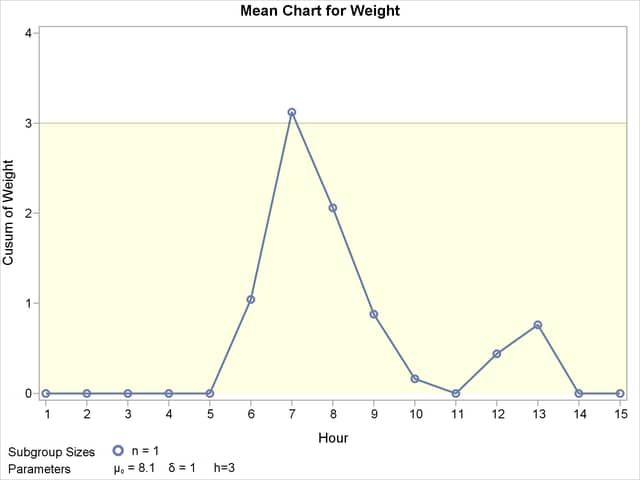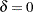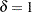The CUSUM Procedure

### Saving Cusum Scheme Parameters

[See CUSONES1 in the SAS/QC Sample Library]This example is a continuation of the previous example that illustrates how to save cusum scheme parameters in a data set specified with the OUTLIMITS= option. This enables you to apply the parameters to future data or to subsequently modify the parameters with a DATA step program.

```ods graphics on;
title 'One-Sided Cusum Analysis';
proc cusum data=Cans;
xchart Weight*Hour /
mu0       = 8.100     /* target mean for process     */
sigma0    = 0.050     /* known standard deviation    */
delta     = 1         /* shift to be detected        */
h         = 3         /* cusum parameter h           */
k         = 0.5       /* cusum parameter k           */
scheme    = onesided  /* one-sided decision interval */
outlimits = cusparm;
label Weight = 'Cusum of Weight';
run;
```

The chart, shown in Figure 6.10, is similar to the one in Figure 6.6 but is created by using ODS Graphics because the ODS GRAPHICS ON statement is specified before the PROC CUSUM statement.

Figure 6.10 One-Sided Cusum Scheme with Decision IntervalThe OUTLIMITS= data set is listed in Figure 6.11.

Figure 6.11 Listing of the OUTLIMITS= Data Set cusparm
 One-Sided Cusum Analysis

Obs _VAR_ _SUBGRP_ _TYPE_ _LIMITN_ _H_ _K_ _SCHEME_ _MU0_ _DELTA_ _MEAN_ _STDDEV_ _ARLIN_ _ARLOUT_
1 Weight Hour STANDARD 1 3 0.5 ONESIDED 8.1 1 8.09747 0.05 117.596 6.40391

The data set contains one observation with the parameters for process Weight. The variables _TYPE_, _H_, _K_, _MU0_, _DELTA_, and _STDDEV_ save the parameters specified with the options SCHEME=, H=, K=, MU0=, DELTA=, and SIGMA0=, respectively. The variable _MEAN_ saves an estimate of the process mean, and the variable _LIMITN_ saves the nominal sample size. The variables _ARLIN_ and _ARLOUT_ save the average run lengths forand for.

The variables _VAR_ and _SUBGRP_ save the process and subgroup-variable. The variable _TYPE_ is a bookkeeping variable that indicates whether the value of _STDDEV_ is an estimate or a standard value.